Loading 2022 NFL Defensive Rookie of the Year Futures...
2022 NFL Defensive Rookie of the Year Odds
+200
2 to 1Devin Lloyd
16.4% implied probability

+500
5 to 1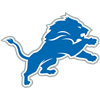Aidan Hutchinson
8.2% implied probability

+750
7.5 to 1Travon Walker
5.8% implied probability

+900
9 to 1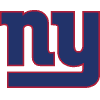Kayvon Thibodeaux
4.9% implied probability

+1200
12 to 1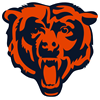Jaquan Brisker
3.8% implied probability

+1200
12 to 1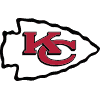George Karlaftis
3.8% implied probability

+1200
12 to 1Malcolm Rodriguez
3.8% implied probability

+1400
14 to 1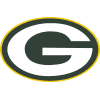Quay Walker
3.3% implied probability

+1500
15 to 1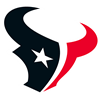Jalen Pitre
3.1% implied probability

+1800
18 to 1Sauce Gardner
2.6% implied probability

+1800
18 to 1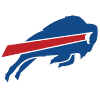Kaiir Elam
2.6% implied probability

+1800
18 to 1Jermaine Johnson
2.6% implied probability

+1800
18 to 1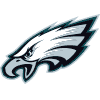Jordan Davis
2.6% implied probability

+2200
22 to 1Derek Stingley
2.1% implied probability

+2500
25 to 1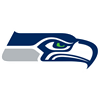Tariq Woolen
1.9% implied probability

+3000
30 to 1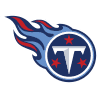Roger McCreary
1.6% implied probability

+3500
35 to 1Jaylen Watson
1.4% implied probability

+3500
35 to 1Boye Mafe
1.4% implied probability

+4000
40 to 1Trent McDuffie
1.2% implied probability

+4000
40 to 1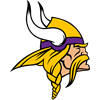Andrew Booth
1.2% implied probability

+4000
40 to 1Jack Jones
1.2% implied probability

No changes have been recorded yet.
+4000
40 to 1Kyle Hamilton
1.2% implied probability

+5000
50 to 1Kerby Joseph
1.0% implied probability

+5000
50 to 1Coby Bryant
1.0% implied probability

+5000
50 to 1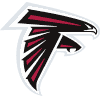Arnold Ebiketie
1.0% implied probability

+5000
50 to 1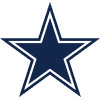Sam Williams
1.0% implied probability

+5000
50 to 1Drake Jackson
1.0% implied probability

+5500
55 to 1Kyler Gordon
0.9% implied probability

+5500
55 to 1Leo Chenal
0.9% implied probability

+6000
60 to 1Logan Hall
0.8% implied probability

+6500
65 to 1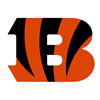Dax Hill
0.7% implied probability

+6500
65 to 1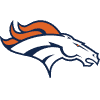Nik Bonitto
0.7% implied probability

+6500
65 to 1Nakobe Dean
0.7% implied probability

+6500
65 to 1DeMarvin Leal
0.7% implied probability

+6500
65 to 1Devonte Wyatt
0.7% implied probability

+7500
75 to 1Troy Andersen
0.6% implied probability

+7500
75 to 1Christian Harris
0.6% implied probability

+8000
80 to 1Chad Muma
0.6% implied probability

+8000
80 to 1Brian Asamoah
0.6% implied probability

+8000
80 to 1Cameron Thomas
0.6% implied probability

+8000
80 to 1Travis Jones
0.6% implied probability

+8000
80 to 1Myjai Sanders
0.6% implied probability

+8000
80 to 1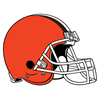Martin Emerson
0.6% implied probability

+8000
80 to 1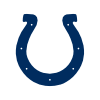Nick Cross
0.6% implied probability

+9000
90 to 1Josh Paschal
0.5% implied probability

+9000
90 to 1Bryan Cook
0.5% implied probability

+10000
100 to 1Kingsley Enagbare
0.5% implied probability

+10000
100 to 1Marcus Jones
0.5% implied probability

+10000
100 to 1Darrian Beavers
0.5% implied probability

+10000
100 to 1Brandon Smith
0.5% implied probability

+10000
100 to 1Cam Taylor-Britt
0.5% implied probability

+10000
100 to 1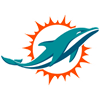Channing Tindall
0.5% implied probability

+15000
150 to 1Mykael Wright
0.3% implied probability

+15000
150 to 1Derion Kendrick
0.3% implied probability

+15000
150 to 1DeAngelo Malone
0.3% implied probability

+15000
150 to 1Josh Jobe
0.3% implied probability

+15000
150 to 1Haskell Garrett
0.3% implied probability

+15000
150 to 1Jack Sanborn
0.3% implied probability

+15000
150 to 1Mario Goodrich
0.3% implied probability

+15000
150 to 1Perrion Winfrey
0.3% implied probability